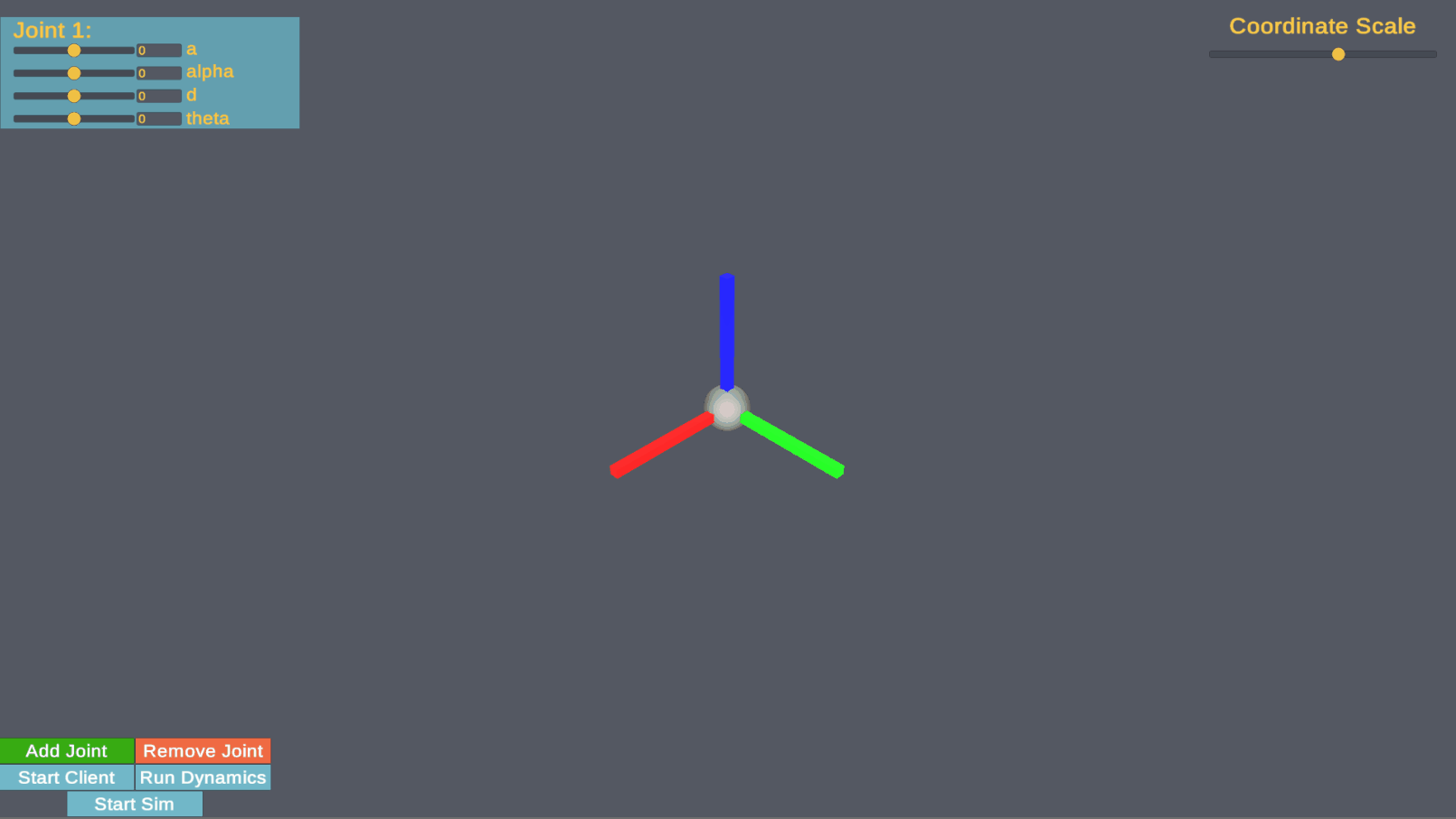Back to Portfolio Home

Summary - This builds off of the DH visualization program using Unity, and extends it to find closed-form dynamic equations and can simulate them given valid mass properties, all from a GUI. This can speed up the design process by enabling quick iteration solely based on DH parameters and mass properties to understand what dynamic behaviours can be expected.

# Generalized Dynamics and Simulation

The goal of this project for me was to do a deep dive into the dynamics and simulation of robots. I have had to setup several simulations, through both classes and research, but wanted to get a better understanding for the underlying principles. All of the code for this project is available on the github page. You can see the full result of this project in the gif below.## Goals

The goals of this project were

• Generate and visualize kinematics from dh parameters in realtime
• Automatically calculate governing equations of motion of a system from dh parameters
• Simulate the developed equations of motion in realtime
• Bonus: Be able to do this all in one session

# DH Parameter Visualization

The first part of this project was to provide an interactive interface for someone to explore DH convention in an intuitive way. The interface lets you slide or input DH parameters to understand how the resulting coordinate systems change. I made something similar in matlab when I took my robotics course, and I think this tool would be especially useful to explain how this works in practice. I made the interface using Unity, and the equations that govern the visualization are from Introduction to Robotics Third Edition by John Craig.

# Automatic Generate Equations of Motion

The second goal of this project was to automatically calculate the equations of motion for the system given the DH parameters. This can be done given explicit equations also found in Introduction to Robitcs by Craig. This was implemented in Python using the Scipy toolkit. The resulting equations were then converted into c++ files that could calculate the EOM of a robot in realtime. To get the properties of the robot, I setup a json file with mass/inertia properties that describe the robot.

# Simulate EOMs in realtime

Once those equations of motion were developed, I used system commands to get cmake to compile the code without leaving the program. Once that was done, I loaded the created dll into the unity simulation, and added open-loop torque controllers at each joint that could again be controlled using sliders to validate that the dynamics were generated correctly.